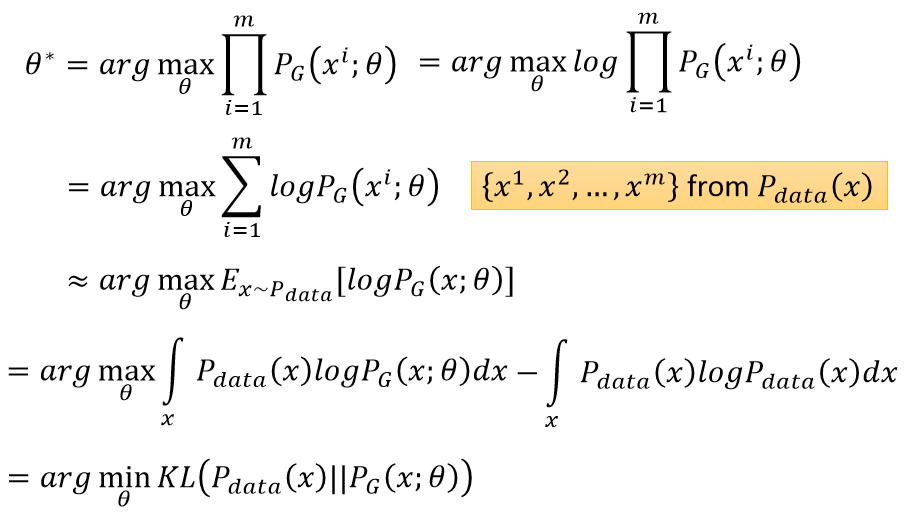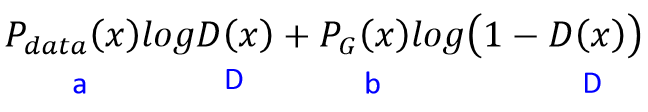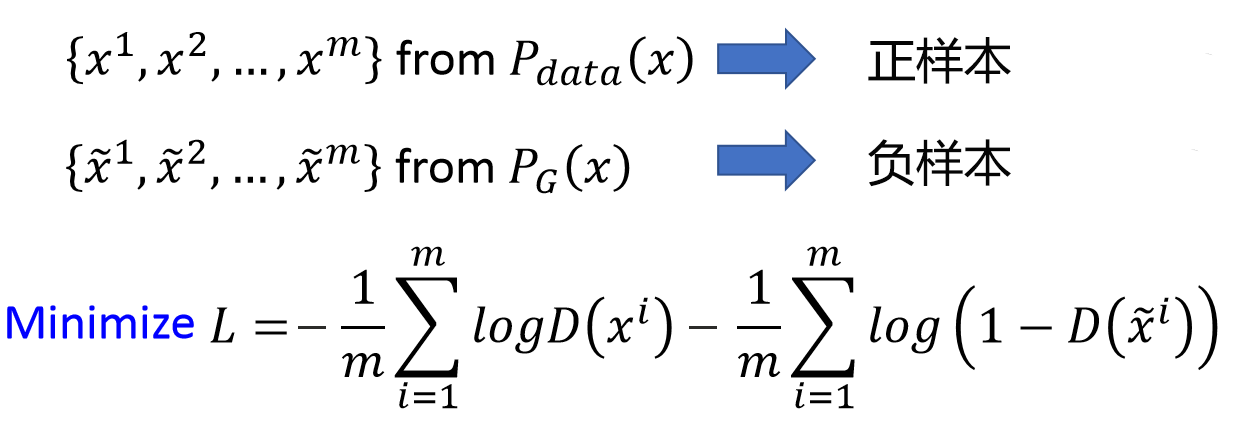# 生成对抗网络

## 解析原版GAN的完整理论推导与实现

### 生成对抗网络基本概念

$\min _ { G } \max _ { D } V ( D ,G ) = \mathbb { E } _ { x \sim p _ { \operatorname{data} } ( x ) } ( \log D ( x ) ] + \mathbb { E } _ { z \sim p _ { z } ( z ) } [ \log ( 1- D ( G ( z ) ) ) ]$### 概念与过程的形式化

#### 最优化问题

$E _ { x \sim p _ { data } } ( x ) \log ( D ( x ) )$

$D ( x ) = 1\text{ when } x \sim p _ { d a t a } ( x )$

$E _ { z \sim p _ { z } ( z ) } \log ( 1- D ( G ( z ) ) )$

$E_{ x \sim p _ { d a t a } ( x )} \log ( D ( x ) ) + E _ { z \sim p _ { z } ( z ) }\log ( 1- D ( G ( z ) ) )$

$V ( G ,D ) : = E _ { x \sim p _ { d a t a } ( x ) } \log ( D ( x ) ) + E _ { z \sim p _ { z } ( z ) } \log ( 1- D ( G ( z ) ) )$

$D _ { G } ^\star = \operatorname{arg} \max _ { D } V ( G ,D )$

$\min _ { G } \max _ { D } V ( D ,G ) = \mathbb { E } _ { x \sim p _ { \text{data} } ( x ) } [ \log D ( x ) ] + \mathbb { E } _ { z \sim p _ { z } } ( z ) [ \log ( 1- D ( G ( z ) ) ) ]$

$G ^\star= \operatorname{arg} \min _ { G } V \left( G ,D _ { G } ^\star \right)$

### 理论推导

#### KL 散度

$H ( x ) = \mathbb { E } _ { x \sim P } [ I ( x ) ] = - \mathbb { E } _ { x \sim P } [ \log P ( x ) ]$

$D _ { K L } ( P | Q ) = \mathbb { E } _ { x \sim P } \left[ \log \frac { P ( x ) } { Q ( x ) } \right] = \mathbb { E } _ { x \sim P } [ \log P ( x ) - \log Q ( x ) ]$

KL 散度有很多有用的性质，最重要的是它是非负的。KL 散度为 0 当且仅当 P 和 Q 在离散型变量的情况下是相同的分布，或者在连续型变量的情况下是 『几乎处处』 相同的。因为 KL 散度是非负的并且衡量的是两个分布之间的差异，它经常 被用作分布之间的某种距离。然而，它并不是真的距离因为它不是对称的：对于某 些 P 和 Q，D_KL(P||Q) 不等于 D_KL(Q||P)。这种非对称性意味着选择 D_KL(P||Q) 还是 D_KL(Q||P) 影响很大。

$L = \prod _ { i = 1} ^ { m } P _ { G } \left( x ^ { i } ; \theta \right)$$\int _ { x } P _ { \operatorname{data} } ( x ) \log P _ { G } ( x ,\theta ) - P _ { d a t a } ( x ) \log P _ { d a t a } ( x ) d x = \int _ { x } P _ { d a t a } ( x ) \log \frac { P _ { G } ( x ,\theta ) } { P _ { d a t a } ( x ) }$

#### 推导存在的问题

$E _ { z \sim p _ { z } ( z ) } \log ( 1- D ( G ( z ) ) ) = E _ { x \sim p _ { G } ( x ) } \log ( 1- D ( x ) )$

$\left.\begin{array} { l } { \int _ { x } p _ { d a t a } ( x ) \log D ( x ) d x + \int _ { z } p ( z ) \log ( 1- D ( G ( z ) ) ) dz } \ { = \int _ { x } p _ { d a t a } ( x ) \log D ( x ) + p _ { G } ( x ) \log ( 1- D ( x ) ) \text{d} x } \end{array} \right.$

#### 最优判别器

$V ( G ,D ) = \int _ { x } p _ { data} ( x ) \log ( D ( x ) ) + p _ { g } ( x ) \log ( 1- D ( x ) ) d x$$f ( y ) = a \log y + b \log ( 1- y )$

$f ^ { \prime } ( y ) = 0\Rightarrow \frac { a } { y } - \frac { b } { 1- y } = 0\Rightarrow y = \frac { a } { a + b }$

$f ^ { \prime \prime } \left( \frac { a } { a + b } \right) = - \frac { a } { \left( \frac { a } { a + b } \right) ^ { 2} } - \frac { b } { 1- \left( \frac { a } { a + b } \right) ^ { 2} } < 0$

\left.\begin{aligned} V ( G ,D ) & = \int _ { x } p _ { d a t a } ( x ) \log D ( x ) + p _ { G } ( x ) \log ( 1- D ( x ) ) d x \leq \int _ { x } \max_y p _ { d a t a } ( x ) \log y + p _ { G } ( x ) \log ( 1- y ) \text{d} x \end{aligned} \right.

#### 最优生成器

$D _ { G } ^ { * } = \frac { p _ { d a t a } } { p _ { d a t a } + p _ { G } } = \frac { 1} { 2}$

「当且仅当 $P_G=P_{data}$，训练标准 C(G)=max V(G,D) 的全局最小点可以达到。」

$V \left( G ,D _ { G } ^ { * } \right) = \int _ { x } p _ { d a t a } ( x ) \log \frac { 1} { 2} + p _ { G } ( x ) \log \left( 1- \frac { 1} { 2} \right) d x$

$V \left( G ,D _ { G } ^ { * } \right) = - \log 2\int _ { x } p _ { G } ( x ) \text{d} x - \log 2\int _ { x } p _ { d a t a } ( x ) \text{d} x = - 2\log 2= - \log 4$

$C ( G ) = \int _ { x } p _ { d a t a } ( x ) \log \left( \frac { p _ { d a t a } ( x ) } { p _ { G } ( x ) + p _ { d a t a } ( x ) } \right) + p _ { G } ( x ) \log \left( \frac { p _ { G } ( x ) } { p _ { G } ( x ) + p _ { d a t a } ( x ) } \right) \text{d} x$

\left.\begin{aligned} C ( G ) & = \int _ { x } ( \log 2- \log 2) p _ { d a t a } ( x ) + p _ { d a t a } ( x ) \log ( \frac { p _ { d a t a } ( x ) } { p _ { G } ( x ) + p _ { d a t a } ( x ) } ) + ( \log 2- \log 2) p _ { G } ( x ) + p _ { G } ( x ) \log ( \frac { p _ { G } ( x ) } { p _ { G } ( x ) + p _ { d a t a } ( x ) } ) \text{d} x \end{aligned} \right.

$\left.\begin{array} { c } { C ( G ) = - \log 2\int _ { x } p _ { G } ( x ) + p _ { d a t a } ( x ) d x } { + \int _ { p } p _ { d a t a } ( x ) \left( \log 2+ \log \left( \frac { p _ { d a t a } ( x ) } { p _ { G } ( x ) + p _ { d a t a } ( x ) } \right) \right) }{ + p _ { G } ( x ) \left( \log 2+ \log \left( \frac { p _ { G } ( x ) } { p _ { G } ( x ) + p _ { d a t a } ( x ) } \right) \right) \text{d} x } \end{array} \right.$

$- \log 2\int _ { x } p G ( x ) + p _ { d a t a } ( x ) \text{d} x = - \log 2( 1+ 1) = - 2\log 2= - \log 4$

\left.\begin{aligned} \log 2+ \log ( \frac { p _ { d a t a } ( x ) } { p _ { G } ( x ) + p _ { d a t a } ( x ) } ) & = \log ( 2\frac { p _ { d a t a } ( x ) } { p _ { G } ( x ) + p _ { d a t a } ( x ) } ) = \log ( \frac { p _ { d a t a } ( x ) } { ( p _ { G } ( x ) + p _ { d a t a } ( x ) ) / 2} \end{aligned} \right.

\left.\begin{aligned} C ( G ) & = - \log 4+ \int _ { x } p _ { d a t a } ( x ) \log ( \frac { p _ { d a t a } ( x ) } { ( p _ { G } ( x ) + p _ { d a t a } ( x ) ) / 2} ) d x + \int _ { x } p _ { G } ( x ) \log ( \frac { p _ { G } ( x ) } { ( p _ { G } ( x ) + p _ { d a t a } ( x ) ) / 2} ) d x \end{aligned} \right.

$C ( G ) = - \log 4+ K L \left( p _ { d a t a } | \frac { p _ { d a t a } + p _ { G } } { 2} \right) + K L \left( p _ { G } | \frac { p _ { d a t a } + p _ { G } } { 2} \right)$

KL 散度是非负的，所以我们马上就能看出来 -log4 为 C(G) 的全局最小值。

$\left.\begin{array} { r l } { \text{JSD} ( P | Q ) = \frac { 1} { 2} D ( P | M ) + \frac { 1} { 2} D ( Q | M ) } \ { M = \frac { 1} { 2} ( P + Q ) } \end{array} \right.$

JS 散度的取值为 0 到 log2。若两个分布完全没有交集，那么 JS 散度取最大值 log2；若两个分布完全一样，那么 JS 散度取最小值 0。

$C ( G ) = - \log 4+ 2\cdot J S D \left( p _ { d a t a } | p _ { G } \right)$

#### 重述训练过程

1.参数优化过程

$\theta _ { G } \leftarrow \theta _ { G } - \eta \partial L ( G ) / \partial \theta _ { G }$

• 给定 $G_0$，最大化 $V(G_0,D)$ 以求得 $D^\star$，即 $max[JSD(P_{data}(x)||P_{G_0}(x)]$；
• 固定 $D_0^\star$，计算 $θ_{G_1} ← θ_{G_0} −η(𝜕V(G,D_0^\star) /𝜕θ_G)$ 以求得更新后的 $G_1$；
• 固定 $G_1$，最大化 $V(G_1,D_0^\star)$ 以求得 D_1*，即 $max[JSD(P_{data}(x)||P_{G_1}(x)]$；
• 固定 $D_1^\star$，计算 $θ_{G_2} ← θ_{G_1} −η(𝜕V(G,D_1^\star) /𝜕θ_G)$ 以求得更新后的 $G_2$；
• 。。。

2.实际训练过程

$Maximize\ \ \tilde { V } = \frac { 1} { m } \sum _ { i = 1} ^ { m } \log D \left( x ^ { i } \right) + \frac { 1} { m } \sum _ { i = 1} ^ { m } \log \left( 1- D \left( \tilde { x } ^ { i } \right) \right)$• 从真实数据分布 P_data 抽取 m 个样本
• 从先验分布 P_prior(z) 抽取 m 个噪声样本
• 将噪声样本投入 G 而生成数据${\tilde{x}^1,\tilde{x}^2, \dots,\tilde{x}^m},\tilde{x}^1=G(z^i)$ ，通过最大化 V 的近似而更新判别器参数θ_d，即极大化 $\tilde { V } = \frac { 1} { m } \sum _ { i = 1} ^ { m } \log D \left( x ^ { i } \right) + \frac { 1} { m } \sum _ { i = 1} ^ { m } \log \left( 1- D \left( \tilde { x } ^ { i } \right) \right)$，且判别器参数的更新迭代式为 $\theta _ { d } \leftrightarrow \theta _ { d } + \eta \nabla \tilde { V } \left( \theta _ { d } \right)$。

• 从先验分布 P_prior(z) 中抽取另外 m 个噪声样本 ${z^1,…,z^m}$
• 通过极小化 $\tilde{V}$ 而更新生成器参数 $θ_g$，即极小化 $\tilde { V } = \frac { 1} { m } \sum _ { i = 1} ^ { m } \log \left( 1- D \left( G \left( z ^ { i } \right) \right) \right)$，且生成器参数的更新迭代式为 $\theta _ { g } \leftrightarrow \theta _ { g } - \eta \nabla \tilde { V } \left( \theta _ { g } \right)$。

### 实现

#### 训练

• 加载 MNIST 数据
• 将数据分割为训练与测试集，并赋值给变量
• 设置训练模型的超参数
• 编译模型的训练过程
• 在每一次迭代内，抽取生成图像与真实图像，并打上标注
• 随后将数据投入到判别模型中，并进行训练与计算损失
• 固定判别模型，训练生成模型并计算损失，结束这一次迭代